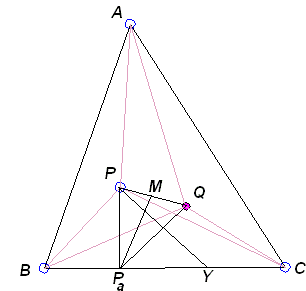### Droz-Farny Circles

Arnold Droz-Farny (1865-1912), a discoverer of a remarkable line now bearing his name, has also established the congruence of two related circles:

### This applet requires Sun's Java VM 2 which your browser may perceive as a popup. Which it is not. If you want to see the applet work, visit Sun's website at https://www.java.com/en/download/index.jsp, download and install Java VM and enjoy the applet.

 What if applet does not run?
 Suppose that P and Q are any pair of isogonal conjugates in ΔABC and that Pa, Pb, and Pc are the feet of perpendiculars from P to the sides of the triangle. Assume also that the circles with centers Pa, Pb, and Pc are drawn to pass through Q. Then the three pairs of points these circles determine on the side line of ΔABC will always lie on the circle with center P. A circle with center Q is obtained in a similar manner. The two circles always have the same radius.

Droz-Farny has proved the theorem for a special pair of isogonal conjugates: O (circumcenter) and H (orthocenter) of a triangle, but it is as easily proven in a more general case.

The proof is based on a metric property of medians in a triangle, that is convenient to write as

 b2 + c2 = 2ma2 + a2/2,

which we apply to the median PaM in triangle PaPQ.Let Y be one of the intersections of BC with the circle through Q centered at Pa, so that

 PaY = PaQ.

In the triangle PaPQ, the Pythagorean theorem gives:

(1) .
 PY2 = PPa2 + PaY2 = PPa2 + PaQ2 = 2·PaM2 + PQ2/2

In the last expression, PaM is the radius of the pedal circle of P with respect to ΔABC and is, thus, independent of being associated with the side BC. PY, therefore, would be exactly the same if, instead of a circle with center at Pa, we chose circles with centers on sides AB or AC. We conclude that there is a circle centered at P that passes through the six points cut on the sides of the triangle by the circles through Q centered at the feet of perpendiculars from P. But now the same holds with regard to point Q: there is a circle through the six points cut by the circles through P centered at the feet of perpendiculars from Q to the sides. Furthermore, since the pedal circles of a pair of isogonal conjugates coincide, (1) produces the same result for the two circles.

### References

1. R. Honsberger, Episodes in Nineteenth and Twentieth Century Euclidean Geometry, MAA, 1995, pp. 69-72 (2004) 219-224